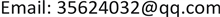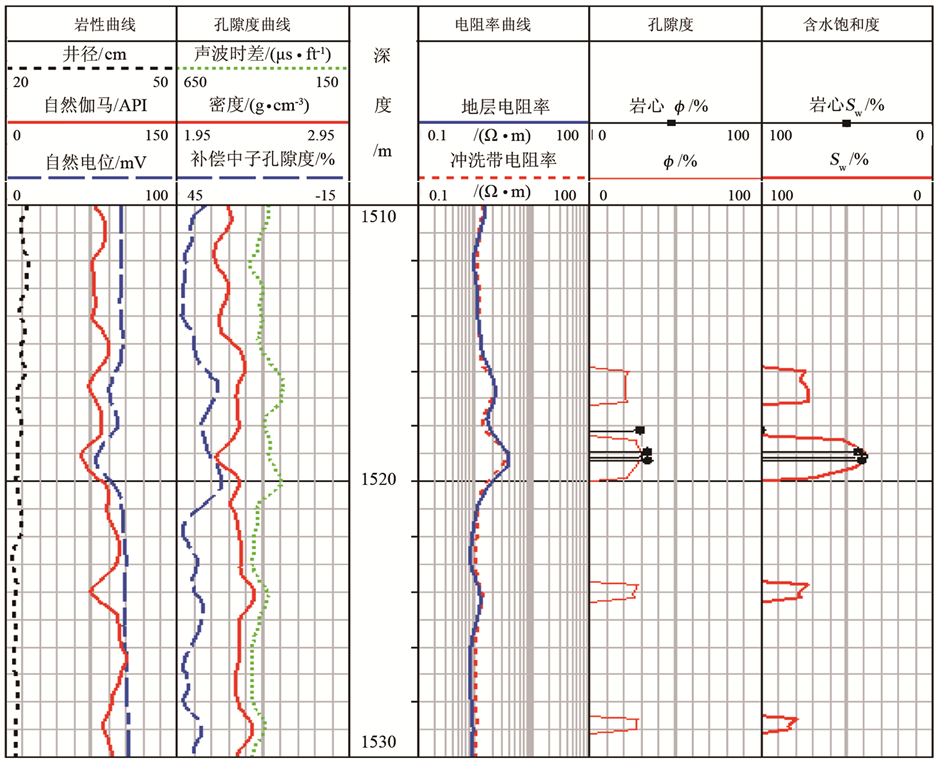﻿ 利用磷虾群觅食优化算法求取阿尔奇公式参数 A Method for Calculating Parameters of Archie Formula Using Krill Foraging Algorithm

Journal of Oil and Gas Technology
Vol.40 No.03(2018), Article ID:25886,6 pages
10.12677/JOGT.2018.403083

A Method for Calculating Parameters of Archie Formula Using Krill Foraging Algorithm

Rengao Jin, Haibin Qiu

Production Logging Center, Logging Co. Ltd., CNPC, Xi’an ShaanxiReceived: Dec. 29th, 2017; accepted: Mar. 29th, 2018; published: Jun. 15th, 2018ABSTRACT

The accurate selection of each parameter value in Archie formula had a great influence on the result of water saturation. In order to solve the problem of insufficient core and rock electricity data in rock electricity test and some areas, it is difficult to get the parameter of Archie formula effectively and accurately. Based on the water saturation test data, physical property data and resistivity data of 30 core samples, the parameters of the Archie formula obtained by using the global searching ability Krill Foraging optimization algorithm were used for obtaining Archie parameters a, b, m, n, which were 0.9931, 1.0308, 2.322 and 2.133 respectively. The water saturation of the other 8 samples was predicted by using the calculation parameters, and the results were compared with those of the core analysis, the average relative error was within 10%, the fitting effect was good. The results show that the krill foraging algorithm is reliable and effective in the estimation of saturation parameters, and has the application prospect, which is beneficial for evaluating the water saturation in reservoirs.

Keywords:Krill Foraging Algorithm, Archie Formula, Parameter Optimization1. 阿尔奇公式

1942年，阿尔奇    通过了对若干取心岩样进行深入研究，提出岩石电阻率与其岩性、孔隙度和含水饱和度的关系，即阿尔奇定律。

1) 饱含地层水的纯净砂岩的电阻率与地层中水的电阻(即砂岩的含水饱和度Sw = 1)成正比，由此得到的比例常数被称作地层因子(一般用F来表示)，得到的公式为阿尔奇第一公式：

$\frac{{\rho }_{0}}{{\rho }_{\text{w}}}=F=\frac{a}{{\varphi }^{m}}$ (1)

2) 当岩石中注入其他流体(油、气)时，砂岩的Sw < 1，该种情况下的岩石电阻率与该岩石完全含水时的电阻率成正比，由此得到的比例系数被称为电阻率指数或电阻率放大系数(一般用I表示)，得到的公式为阿尔奇第二公式：

 $\frac{{\rho }_{\text{t}}}{{\rho }_{0}}=I=\frac{b}{{S}_{\text{w}}^{n}}$ (2)

${S}_{\text{w}}=\sqrt[n]{\frac{ab{\rho }_{\text{w}}}{{\varphi }^{m}{\rho }_{\text{t}}}}$ (3)

2. 磷虾群觅食优化算法原理及适应度函数建立

2.1. 磷虾群觅食优化算法原理

1) 初始化：设置种群规模NP，最大迭代次数Imax，其中磷虾群觅食优化算法构建的拉格朗日模型为：

$\frac{\text{d}{X}_{i}}{\text{d}t}={N}_{i}+{F}_{i}+{D}_{i}$ (4)

2) 适应度值以及当前最优个体计算：评价适应度值，进而确定当前最优个体Xbest

3) 位置更新：根据磷虾群觅食优化算法，计算并更新所有个体的运动矢量，具体运动向量包括受诱导运动向量、觅食行为向量和扩散行为向量：

${N}_{i}^{\text{new}}={N}^{\mathrm{max}}\left({\alpha }_{i}^{\text{local}}+{\alpha }_{i}^{\text{target}}\right)+{\omega }_{\text{n}}{N}_{i}^{\text{old}}$ (5)

${F}_{i}^{\text{new}}={v}_{\text{f}}\left({\beta }_{i}^{\text{food}}+{\beta }_{i}^{\text{best}}\right)+{\omega }_{\text{f}}{F}_{i}^{\text{old}}$ (6)

${D}_{i}^{\text{new}}={D}^{\mathrm{max}}\left(1-\frac{1}{{I}_{\mathrm{max}}}\right)\delta$ (7)

4) 位置更新：计算所有个体的运动矢量，根据磷虾群觅食优化算法更新位置。

5) 动态调整当前迭代的搜索域范围[LG, UG]：

$\begin{array}{l}{l}_{\text{K}}^{\text{G}}=\text{min}\left\{{x}_{i,k}|i=1,2,\cdot \cdot \cdot ,{N}_{\text{P}}\right\}\\ {u}_{\text{K}}^{\text{G}}=\text{max}\left\{{x}_{i,k}|i=1,2,\cdot \cdot \cdot ,{N}_{\text{P}}\right\}\end{array}$

6) 若迭代次数I < Imax，则令I = I + 1，转步骤2)；否则，输出最优解X* = Xbest

2.2. 目标函数与适应度函数的建立

$\mathrm{min}f\left(x\right)=\frac{1}{\mathrm{min}\sum _{i=1}^{N}e{\left(i\right)}^{2}}$ (8)

$f\left(a,b,m,n\right)=\frac{1}{\mathrm{min}\sum _{i=1}^{N}{\left({S}_{\text{wi},i}-{S}_{\text{w},i}\right)}^{2}}=\frac{1}{\mathrm{min}{\sum _{i=1}^{N}{\left(\sqrt[n]{\frac{ab{\rho }_{w}}{{\varphi }_{i}^{m}{\rho }_{t,i}}}-{S}_{\text{w},i}\right)}^{2}}_{}^{}}$ (9)

3. 模型实际运用效果分析Table 1. The analysis on the predicted result of SwFigure 1. The diagram of saturation evaluation of a well

4. 结语

 雍世和, 张超谟. 测井数据处理与综合解释[M]. 东营: 中国石油大学出版社, 2007.

 Archie, J.G. and Archie, G. (1942) The Electrical Resistivity Logs as an Aid in Determining Some Reservoir Characteristic. Transactions of the AIME, 52, 354-358. https://doi.org/10.2118/942054-G

 Rogers, S.J., Fans, J.H., Karr, E., et al. (1992) Determination of Lithology from Well Logs Using a Neural Network. AAPG Bulletin, 76, 731-739.

 朱林奇, 张冲, 周雪晴, 等. 融合深度置信网络与与核极限学习机算法的核磁共振测井储层渗透率预测方法[J]. 计算机应用, 2017, 37(10): 3034-3038.

 闫桂京, 潘保芝. 遗传算法在估计测井解释参数方面的应用[J]. 物探化探计算技术, 2001, 23(1): 43-46, 67.

 朱林奇, 张冲. 谱聚类-Adaboost集成数据挖掘算法在岩性识别中的应用[J]. 中国科技论文, 2016, 11(5): 545-550.

 文浩. 遗传模拟退火算法在阿尔奇公式参数估计中的应用[J]. 断块油气田, 2008, 15(1): 105-107.

 牛步能. 利用雨林算法求取阿尔奇公式参数的方法[J]. 长江大学学报(自科版), 2016, 13(20): 32-35.

 朱林奇, 张冲, 魏旸, 等. 基于改进雨林模糊神经网络模型的页岩储层总有机碳含量评价方法[J]. 高校地质学报, 2016, 22(4): 716-723.

 Gandomi, A.H. and Alavi, A.H. (2012) Krill Herd: A New Bio-Inspired Optimization Algorithm. Communications in Nonlinear Science and Numerical Simulation, 17, 4831-4845. https://doi.org/10.1016/j.cnsns.2012.05.010

 王磊, 张汉鹏, 张东宁. 基于对立搜索和混沌变异的磷虾群觅食优化算法[J]. 控制与决策, 2015(9): 1617-1622.

 Mandal, B., Roy, P.K. and Mandal, S. (2014) Economic Load Dispatch Using Krill Algorithm. International Journal of Electrical Power and Energy Systems, 57, 1-10. https://doi.org/10.1016/j.ijepes.2013.11.016

 Wang, G.G., Guo, L.H., Gandomi, A.H., et al. (2014) Chaotic Krill Herd Algorithm. Information Sciences, 274, 17-34. https://doi.org/10.1016/j.ins.2014.02.123

 Wang, G.G., Gandomi, A.H. and Alavi, A.H. (2014) Stud Krill Herd Algorithm. Neurocomputing, 128, 363-370. https://doi.org/10.1016/j.neucom.2013.08.031

 周雪晴, 张占松, 张超谟, 等. 粗糙集–磷虾群觅食优化算法神经网络在孔隙度预测中的应用[J]. 中国科技论文, 2017, 12(9): 990-998.

 张智, 姜秋喜, 徐梁昊. 磷虾群免疫粒子滤波的机载单站无源定位算法[J]. 火力与指挥控制, 2015, 40(4): 92-97.

[编辑] 龚丹